# Double layer (plasma)

Double layer (plasma)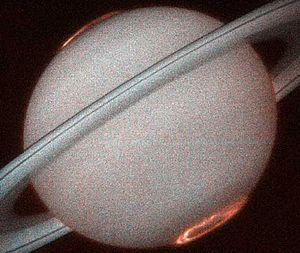Saturnian aurora whose reddish colour is characteristic of ionized hydrogen plasma. Powered by the Saturnian equivalent of (filamentary) Birkeland currents, streams of charged particles from the interplanetary medium interact with the planet's magnetic field and funnel down to the poles. Double layers are associated with (filamentary) currents, and their electric fields accelerate ions and electrons.

A double layer is a structure in a plasma and consists of two parallel layers with opposite electrical charge. The sheets of charge cause a strong electric field and a correspondingly sharp change in voltage (electrical potential) across the double layer. Ions and electrons which enter the double layer are accelerated, decelerated, or reflected by the electric field. In general, double layers (which may be curved rather than flat) separate regions of plasma with quite different characteristics. Double layers are found in a wide variety of plasmas, from discharge tubes to space plasmas to the Birkeland currents supplying the Earth's aurora, and are especially common in current-carrying plasmas. Compared to the sizes of the plasmas which contain them, double layers are very thin (typically ten Debye lengths), with widths ranging from a few millimeters for laboratory plasmas to thousands of kilometres for astrophysical plasmas.

Other names for a double layer are electrostatic double layer, electric double layer, plasma double layers, electrostatic shock (a type of double layer which is oriented at an oblique angle to the magnetic field in such a way that the perpendicular electric field is much stronger than the parallel electric field), space charge layer. In laser physics, a double layer is sometimes called an ambipolar electric field. Double layers are conceptually related to the concept of a 'sheath' (see Debye sheath).

The adopted electrical symbol for a double layer, when represented in an electrical circuit is ────DL────. If there is a net current present, then the DL is oriented with the base of the L in line with direction of current.

An overview of double layers in space, experiment and simulation is given in the introduction of ref.

## Double layer classification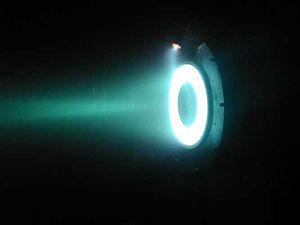Hall effect thruster. The electric fields utilised in plasma thrusters (in particular the Helicon Double Layer Thruster) may be in the form of double layers.

Double layers may be classified in the following ways:

• Weak and strong double layers. The strength of a double layer is expressed as the ratio of the potential drop in comparison with the plasma’s equivalent thermal energy, or in comparison with the rest mass energy of the electrons. A double layer is said to be strong if the potential drop across the layer is greater than the equivalent thermal energy of the plasma’s components. This means that for strong double layers there are four different components to the plasma:
1. The electrons entering at the low potential side of the double layer which are accelerated;
2. The ions entering at the high potential side of the double layer which are accelerated;
3. The electrons entering at the high potential side of the double layer which are decelerated and successively reflected; and
4. The ions which enter the double layer at the low potential side of the double layer which are decelerated and reflected.
Note that in the case of a weak double layer, the electrons and ions entering from the “wrong” side are decelerated by the electric field, however most will not be reflected, as the potential drop is not strong enough.
• Relativistic or nonrelativistic double layers. A double layer is said to be relativistic if the potential drop over the layer is so large that the total gain in energy of the particles is larger than the rest mass energy of the electron. The charge distribution in a relativistic double layer is such that the charge density is located in two very thin layers, and inside the double layer the density is constant at and very low compared to the rest of the plasma. In this respect, the double layer is similar to the charge distribution in a capacitor. As a special case of a relativistic double layer one can consider the vacuum gap at the magnetic polar cap of a pulsar.
• Current carrying and current-free double layers may both occur. Current carrying double layers may be generated by current-driven plasma instabilities which amplify variations of the plasma density. Current-free double layers form on the interface between two plasma regions with different characteristics, and their associated electric field maintains a balance between the penetration of electrons in either direction (so that the net current is low).

## Double layer formation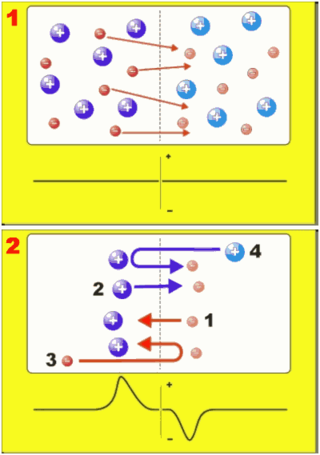Doubler layer formation. Hotter electrons moving into a cooler plasma region (Diagram 1, top) cause a charge imbalance, resulting in a double layer that is able to accelerate electrons across it (Diagram 2, bottom).

There are two different kinds of double layers, which are formed differently:

### Current carrying double layers

Current carrying double layers may arise in plasmas carrying a current. Various instabilities can be responsible for the formation of these layers. One example is the Buneman instability which occurs when the streaming velocity of the electrons (basically the current density divided by the electron density) exceeds the electron thermal velocity of the plasma. Double layers (and other phase space structures) are often formed in the non-linear phase of the instability. One way of viewing the Buneman instability is to describe what happens when the current (in the form of a zero temperature electron beam) has to pass through a region of decreased ion density. In order to prevent charge from accumulating, the current in the system must be the same everywhere (in this 1D model). The electron density also has to be close to the ion density (quasineutrality), so there is also a dip in electron density. The electrons must therefore be accelerated into the density cavity, to maintain the same current density with a lower density of charge carriers. This implies that the density cavity is at a high electrical potential. As a consequence, the ions are accelerated out of the cavity, amplifying the density perturbation. Then there is the situation of a double-double layer, of which one side will most likely be convected away by the plasma, leaving a regular double layer. This is the process in which double layers are produced along planetary magnetic field lines in so-called Birkeland currents.

### Current-free double layers

Current-free double layers occur at the boundary between plasma regions with different plasma properties. We explain how they form (neglecting the ions which are considered solely as a neutralizing background). Consider a plasma divided into two regions by a plane, which has a higher electron temperature on one side than on the other (the same analysis can also be done for different densities). This means that the electrons on one side of the interface have a greater thermal velocity. The electrons may stream freely in either direction, and the flux of electrons from the hot plasma to the cold plasma will be greater than the flux of the electrons from the cold plasma to the hot plasma, because the electrons from the hot side have a greater average speed. Because many more electrons enter the cold plasma than exit it, part of the cold region becomes negatively charged. The hot plasma, conversely, becomes positively charged. Therefore, an electric field builds up, which starts to accelerate electrons towards the hot region, reducing the net flux. In the end, the electric field builds up until the fluxes of electrons in either direction are equal, and further charge build up in the two plasmas is prevented. The potential drop is in fact exactly equal to the difference in thermal energy between the two plasma regions in this case, so such a double layer is a marginally strong double layer.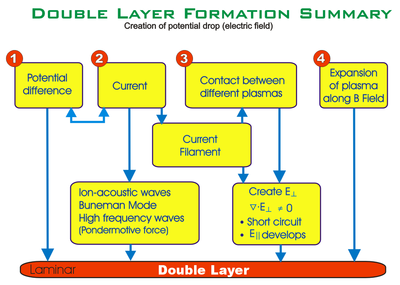Double Layer Formation Summary. Double layers are formed in four main ways

### Double layer formation mechanisms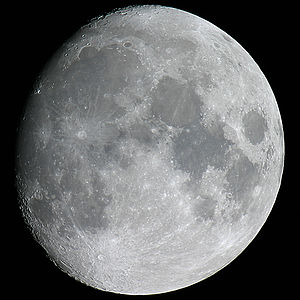The Moon. The prediction of a lunar double layer was confirmed in 2003. In the shadows, the Moon charges negatively in the interplanetary medium.

The details of the formation mechanism depend on the environment of the plasma (e.g. double layers in the laboratory, ionosphere, solar wind, fusion, etc.). Proposed mechanisms for their formation have included:

• 1971: Between plasmas of different temperatures
• 1976: In laboratory plasmas
• 1982: Disruption of a neutral current sheet
• 1983: Injection of non-neutral electron current into a cold plasma
• 1985: Increasing the current density in a plasma
• 1986: In the accretion column of a neutron star
• 1986: By pinches in cosmic plasma regions
• 1987: In a plasma constrained by a magnetic mirror
• 1988: By an electrical discharge
• 1988: Current-driven instabilities (strong double layers)
• 1988: Spacecraft-ejected electron beams
• 1989: From shock waves in a plasma
• 2002: When magnetic field-aligned currents encounter density cavities
• 2003: By the incidence of plasma on the dark side of the Moon's surface

## Features and characteristics of double layers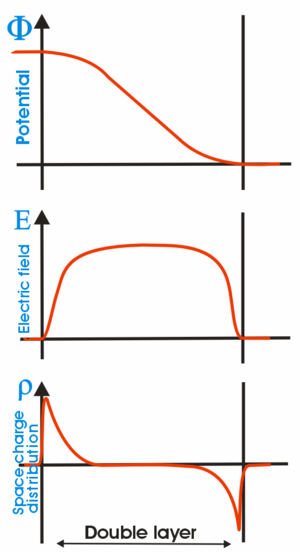Double layer characteristics showing the potential (Φ), electric field (E) and space charge distribution (ρ) across the layer
• Thickness: The production of a double layer requires regions with a significant excess of positive or negative charge, that is, where quasi-neutrality is violated. In general, quasi-neutrality can only be violated on scales of the order of the Debye length. The thickness of a double layer is of the order of ten Debye lengths, which is a few centimeters in the ionosphere, a few tens of meters in the interplanetary medium, and tens of kilometers in the intergalactic medium.
• Particle acceleration: The potential drop across the double layer will accelerate electrons and positive ions in opposite directions. The magnitude of the potential drop determines the acceleration of the charged particles. In strong double layers, this will result in beams or jets of charged particles.
• Particle populations: As described in the formation of double layers, there are four populations of charge particles inside a double layer. Note that in the case of weak double layers not all electrons and ions entering "from the wrong side" will be reflected, and therefore there will also be a population of decelerated electrons and ions.
• Particle flux: For non-relativistic current carrying double layers the electrons comprise the main part of the particle flux. The Langmuir condition states that the ratio of the electron and the ion current across the layer is given by the square root of the mass ratio of the ions to the electrons. For relativistic double layers the current ratio is 1; i.e. equal amounts of current are carried by the electrons and the ions.
• Energy supply: In a certain limit, the voltage drop across a current-carrying double layer is proportional to the total current, and it might be thought of as a resistive element (or load) which absorbs energy in an electric circuit. Anthony Peratt (1991) wrote: "Since the double layer acts as a load, there has to be an external source maintaining the potential difference and driving the current. In the laboratory this source is usually an electrical power supply, whereas in space it may be the magnetic energy stored in an extended current system, which responds to a change in current with an inductive voltage".
• Stability: Double layers in laboratory plasmas may be stable or unstable depending on the parameter regime. Various types of instabilities may occur, often arising due to the formation of beams of ions and electrons. Unstable double layers are noisy in the sense that they produce oscillations across a wide frequency band. A lack of plasma stability may also lead to a dramatic change in configuration often referred to as an explosion (and hence exploding double layer). In one example, the region enclosed in the double layer rapidly expands and evolves. An explosion of this type was first discovered in mercury arc rectifiers used in high-power direct-current transmission lines, where the voltage drop across the device was seen to increase by several orders of magnitude. Double layers may also drift, usually in the direction of the emitted electron beam, and in this respect are natural analogues to the smooth—bore magnetron.) (not to be confused with a unit of magnetic moment, the Bohr magneton, which is created by the "classical circular motion" of an electron around a proton).
 Location Typical Voltage drop Source Ionosphere 102–104V Satellite Solar 109–1011V Estimated Neutron star 1015V Estimated Galactic filament 1017V Estimated
Typical Double Layers
• Magnetised plasmas: Double layers can both form in normal and magnetized plasmas.
• Cellular nature: While double layers are relatively thin, they will spread over the entire cross surface of a laboratory container. Likewise where adjacent plasma regions have different properties, double layers will form and tend to cellularise the different regions.
• Energy transfer: Double layers facilitate the transfer of electrical energy into kinetic energy, dW/dt=I.ΔV where I is the electric current dissipating energy into a double layer with a voltage drop of ΔV. Alfvén points out that the current may well consist exclusively of low-energy particles. Torvén et al. also report that plasma may spontaneously transfer magnetically stored energy into kinetic energy by electric double layers.
• Oblique double layer: An oblique double layer has its electric field not parallel to the background magnetic field; i.e., it is not field-aligned.
• Simulation: Double layers may be modelled using kinetic computer models like particle-in-cell (PIC) simulations. In some cases it is reasonable to treat the plasma as essentially one- or two-dimensional to reduce the computational cost of a simulation.
• Bohm Criterion: A double layer cannot exist under all circumstances. In order to achieve that the electric field vanishes at the boundaries of the double layer, an existence criterion says that there is a maximum to the temperature of the ambient plasma. This is the so-called Bohm criterion. A mathematical description is given in the math section. In the theory of the Debye sheath there is a related but not identical condition also known as the Bohm criterion.
• Bio-physical analogy: A model of plasma double layers has been used to investigate their applicability to understanding ion transport across biological cell membranes. Brazilian researchers have note that "Concepts like charge neutrality, Debye length, and double layer are very useful to explain the electrical properties of a cellular membrane." Plasma physicist Hannes Alfvén also noted that association of double layers with cellular structure, as had Irving Langmuir before him, who coined the named "plasma" after its resemblance to blood cells.

## History of double layersA cluster of double layers forming in an Alfvén wave, about a sixth of the distance from the left. Click for more details

The research of these objects is a relatively young phenomenon. Although it was already known in the 1920s that a plasma has a limited capacity for current maintenance, Irving Langmuir characterized double layers in the laboratory and called these structures double-sheaths. It was not until the 1950s that a thorough study of double layers started in the laboratory (e.g. Schönhuber, 1958).[citation needed] At the moment many groups are working on this topic theoretically, experimentally and numerically. It was first proposed by Hannes Alfvén (the developer of magnetohydrodynamics) that the polar lights or Aurora Borealis are created by accelerated electrons in the magnetosphere of the Earth. He supposed that the electrons were accelerated electrostatically by an electric field localized in a small volume bounded by two charged regions. This so-called double layer would accelerate electrons Earthwards. Many experiments with rockets and satellites have been performed to investigate the magnetosphere and acceleration regions. The first indication for the existence of electric field aligned along the magnetic field (or double layers) in the magnetosphere was by a rocket experiment by McIlwain (1960). Later, in 1977, Forrest Mozer reported that satellites had detected the signature of double layers (which he called electrostatic shocks) in the magnetosphere.

The most definite proof of these structures was obtained by the Viking satellite, which measures the differential potential structures in the magnetosphere with probes mounted on 40m long booms. These probes can measure the local particle density and the potential difference between two points 80m apart. Asymmetric potential structures with respect to 0 V were measured, which means that the structure has a net potential and can be regarded as a double layer. The particle densities measured in such structures can be as low as 33% of the background density. The structures usually have an extent of 100 m (a few tens of Debye lengths). The filling factor of the lower magnetosphere with such solitary structures is about 10%. If one out of 5 such structures has a net potential drop of 1 V then the total potential drop over a region of 5000 km would be more than the 1 kV which is needed for the electrons to create the aurora. Magnetospheric double layers typically have a strength$e\phi_{DL}/k_B T_e \approx 0.1$ (where the electron temperature is assumed to lie in the range ($2 eV \leq k_B T_e \leq 20 eV$) and are therefore weak. The American FAST spacecraft found strong double layers in the auroral acceleration region. Strong Double layers were also found in the return current region by Andersson et al. . The return current region is where electrons move upward from the ionosphere to close the auroral current circuit.

In the laboratory, double layers can be created in different devices. They are investigated in double plasma machines, triple plasma machines, and Q-machines. The stationary potential structures which can be measured in these machines agree very well with what one would expect theoretically. An example of a laboratory double layer can be seen in the figure below, taken from Torvén and Linberg (1980), where we can see how well-defined and confined the potential drop of a double layer in a double plasma machine is. One of the interesting things of the experiment by Torvén and Lindberg (1980) is that not only did they measure the potential structure in the double plasma machine but they also found high-frequency fluctuating electric fields at the high-potential side of the double layer (also shown in the figure). These fluctuations are probably due to a beam-plasma interaction outside the double layer which excites plasma turbulence. Their observations are consistent with experiments on electromagnetic radiation emitted by double layers in a double plasma machine by Volwerk (1993), who, however, also observed radiation from the double layer itself. The power of these fluctuations has a maximum around the plasma frequency of the ambient plasma. It was later found that the electrostatic high-frequency fluctuations near the double layer can be concentrated in a narrow region, sometimes called the hf-spike , on the high potential side of the double layer. Subsequently, both radio emissions, near the plasma frequency, and whistler waves at much lower frequencies were seen to emerge from this region . Similar whistler wave structures were observed together with electron beams near Saturn's moon Enceladus , suggesting the presence of a double layer at lower altitude.

A recent development in double layer experiments is the investigation of so-called stairstep double layers. It has been observed that a potential drop in a plasma column can be split up into different parts. Transitions from a single double layer into two-, three-, or greater-step double layers are strongly sensitive to the boundary conditions of the plasma (Hershkowitz, 1992).[citation needed] These experiments can give us information about the formation of the magnetospheric double layers and their possible role in creating the aurora.

Some scientists have subsequently suggested a role of double layers in solar flares.

## Mathematical description of a double layer

In this section we will take a closer look at the mathematics behind double layers. We first describe a semi-quantitative criterion for the formation of a density dip. We then describe a particularly simple kind of double layer. We then explain how to use the distribution function and the Vlasov-Poisson equation to model more-complex double layers.

### Formation of a density dip

First we will take a look at the generation of a double layer in a current-carrying plasma. In 1968 Alfvén and Carlqvist showed that a density dip in a current carrying plasma can be favorable for the generation of a double layer. In this case we look at the plasma as a combination of two fluids, the moving electron fluid and the immobile ion fluid which acts as a neutralizing background. The electron fluid is treated as an essentially zero temperature beam and the ions are assumed to be collisional, and possess some finite temperature.

The density dip in the plasma (of both electrons and ions) will cause an electric field to be generated in order to keep the current density at the same level; i.e., electrons are accelerated in the decreasing part into the dip and decelerated in the increasing part out of the dip. However, this electric field will also have an influence on the first as immobile assumed ions. These ions will be driven out of the density dip, increasing it, and thereby increasing the electric field. When all ions are gone, the electric field has reached its maximum value over the dip. Note that we then have a double-double layer (increasing and decreasing electric field), and one side needs to be transported away.

We will use the quasi-static, non-relativistic description of this mechanism, which is governed by the continuity equation and the momentum equation:$\frac{\partial}{\partial x} (n_e v_e) = 0,$$m_e v_e \frac{\partial v_e}{\partial x} = - e E.$

Combining these two equations we get an expression for the electric field dependent on the electron density:$E = - \frac{\partial}{\partial x} \left(\frac{m_e j_e^2}{2 n_e^2e^3}\right)$ where je = − neeve the electron current density. The ions will experience an outward force due to this electric field, with$F = e n_i E = - \frac{\partial}{\partial x}(m_e n_e v_e^2)$. Only when the force of the electric field can overcome the force by the ion pressure gradient can the evacuation of the density dip take place. Comparing the two forces (pressure and electric) assuming a quasi-neutral, thermal plasma shows after integration that this can only happen when$k_B T_i < m_e v_e^2$. This happens to be the Bohm criterion for the stability of a double layer (more below).

### Current-carrying double layers formed by single, zero temperature beams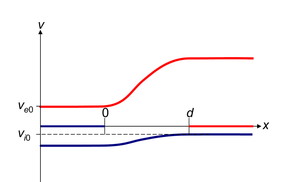The electron (red) and ion (blue) distributions in phase (velocity and position) space for the double layer described in this section. There are two beam-like (zero temperature) components for each species. The ion velocity is not to scale: it is exaggerated for the purposes of visualisation.

We consider how a single zero-temperature beam of ions and a single zero-temperature beam of electrons, together with a trapped, zero-velocity ion component, and a trapped, zero-velocity electron component, may form a particular class of double layer. The trapped components are referred to as the 'ambient plasma' and will later be allowed to have finite temperature.

We use Poisson's equation and the conservation of momentum and number density to analyse the structure of these double layers, in the 1D, time-independent limit. We are looking for double-layerlike solutions, where there is a well localised region with a potential gradient, outside of which the electric field is zero. The region can be divided into the interval inside the double layer, where there is only one ion component and one electron component, but there is a finite field, and the outside region, where the electric field is zero. For the moment, we need only consider the inside region and the densities and velocities associated with the beams inside the layer.

The electron beam component is streaming with positive velocity ve(x) (to the right), and the ion beam is streaming with negative velocity vi(x) (to the left). Here, the conservation of particle energy means that$v_{\alpha}^2(x)/2 m + q_{\alpha} \phi(x)$ is a constant, and the conservation of particle number means that the current jα = qnα(x)vα(x) is also a constant.$|v_{\alpha}(\phi_{\alpha})| = \sqrt{v_{\alpha,0}^2 + \frac{2 e \phi_{\alpha}}{m_{\alpha}}},$$n_{\alpha}(\phi_{\alpha}) = n_0 v_{\alpha,0} v_{\alpha}^{-1}(\phi_{\alpha}),$

where ϕe = ϕ and ϕi = ϕDL − ϕ. Here n0 and vα,0 are respectively the electron (and ion) density and particle drift speed at the low (high) potential side of the double layer.

Now we use Poisson’s equation to obtain the maximal current through the double layer, as a function of the potential drop, the fraction of current carried by the ions as compared to the electrons and a temperature limit for the ambient plasma. We chose ϕ(x = 0) = 0 and ϕ(x = d) = ϕDL, with d the thickness of the double layer.$\rho_e = \frac{j_e}{v_e}, \rho_i = \frac{j_i}{v_i}.$

Thus we can write Poisson’s equation in the region inside the double layer as$- \frac{1}{4 \pi} \frac{\partial^2 \phi}{\partial x^2} = \frac{j_i}{ \sqrt{ v_{i,0}^2 + 2 e (\phi_{DL}-\phi)/m_i } } - \frac{j_e}{ \sqrt{v_{e,0}^2 + 2 e \phi / m_e } }.$

Introducing an integration factor dϕ / dx at both sides and integrating over x at the left hand side and over ϕ on the right hand side the first integration leads to the square of the electric field (dϕ / dx)2. The assumption that there is no electric field outside the double layer then leads to the ‘’Langmuir condition’’ for non-relativistic double layers:$\frac{j_e}{j_i} = \frac{m_i}{m_e}.$

For this double layer (in a hydrogen plasma) the electron current dominates the ion current by a factor of$\sqrt{1836}$. (Note that for the same theory for ultra-relativistic double layers gives this fraction equal to 1). Further integration, as done by Raadu (1989), then leads to the Langmuir-Child relation:$j d^2 = (j_e + j_i) d^2 = \left( 1 + \sqrt{\frac{m_e}{m_i}}\right) \frac{C_0}{9} \sqrt{\frac{2e}{m_e}} \phi_{DL}^{1.5},$

where C0 is expressed in terms of the elliptical integrals E and K:$C_0 = 2^{-1.5} (2 \sqrt{2} E ( \sin\pi/8) - (1 + 2\sqrt{2}K(\sin\pi/8))^2 \approx 1.86518.$

If we now allow the ambient plasma to be at finite temperature we have to take into account reflected particles more carefully and examine how far they can penetrate into the repulsive electric field. We describe the ambient plasma by a Boltzmann distribution over the double layer:$n_{\alpha,R} = n_{\alpha,0} e^{- \frac{e \phi_{\alpha}}{k_B T_{\alpha}}}.$

The densities of the reflected particles are now added to Poisson’s equation. In order that the particles in the 'ambient plasma' be truly trapped we require that their temperature be lower than the double layer potential. This can be seen in terms of the restriction that the potential and the electric field have to vanish at the boundaries of the double layer. The precise condition is known as the ‘’Bohm criterion’’:$m_e v_e^2 > k_B T_i.$

A double layer of this type cannot form if this criterion is not met. This is the same condition under which a double layer can be formed by an ion density dip (or equivalently, for instability to parallel wavemodes like the ion acoustic or Buneman instability) as discussed before.

### The Vlasov–Poisson equation

In general the plasma distributions near a double layer are necessarily strongly non-Maxwellian, and therefore inaccessible to fluid models. In order to analyse double layers in full generality, the plasma must be described using the particle distribution function$f_{\alpha}(\vec{x},t;\vec{v})$, which describes the number of particles of species α having approximately the velocity$\vec{v}$ near the place$\vec{x}$ and time t.

The Vlasov-Poisson equations give the time-dependent interaction of a plasma (described using the particle distribution) with its self-consistent electric field. The Vlasov-Poisson equations are a combination of the Vlasov equation for each species α (we take the nonrelativistic zero-magnetic field limit):$\frac{\partial}{\partial t} f_\alpha + \vec{v} \cdot \frac{\partial}{\partial \vec{x}} f_\alpha + \frac{q_\alpha \vec{E}}{m_\alpha} \cdot \frac{\partial}{\partial \vec{v}} f_\alpha = 0,$

and Poisson’s equation for self-consistent electric field:$\nabla \cdot \vec{E} = -\frac{\partial^2\phi}{\partial x^2} = 4 \pi \rho.$

Here qα is the particle’s electric charge, mα is the particle’s mass,$\vec{E}(\vec{x},t)$ is the electric field,$\phi(\vec{x}, t)$ the electric potential and ρ is the electric charge density.

## Footnotes

1. ^ Astronomy Picture of the Day: 23 December 2001 (NASA)
2. ^ Isbell, J.; Dessler, A. J.; Waite, J. H. "Magnetospheric energization by interaction between planetary spin and the solar wind" (1984) Journal of Geophysical Research, Volume 89, Issue A12, pp. 10715–10722
3. ^ Theisen, William L. "Langmuir Bursts and Filamentary Double Layers in Plasmas." (1994) Ph.D Thesis U. of Iowa, 1994
4. ^ Deverapalli, C. M.; Singh, N.; Khazanov, I. "Filamentary Structures in U-Shaped Double Layers" (2005) American Geophysical Union, Fall Meeting 2005, abstract #SM41C-1202
5. ^ Borovsky, Joseph E. "Double layers do accelerate particles in the auroral zone" (1992) Physical Review Letters (ISSN 0031-9007), vol. 69, no. 7, Aug. 17, 1992, pp. 1054–1056
6. ^ Temerin, M.; Mozer, F. S., "Double Layers Above the Aurora" (1987) NASA Conference Publication, #2469
7. ^ Block, L. P. "A double layer review" (1978) Astrophysics and Space Science, vol. 55, no. 1, May 1978, pp. 59–83
8. ^ Bulgakova, Nadezhda M. et al., "Double layer effects in laser-ablation plasma plumes", Physical Review E (Statistical Physics, Plasmas, Fluids, and Related Interdisciplinary Topics), Volume 62, Issue 4, October 2000, pp. 5624–5635
9. ^ Double Layers in Astrophysics, NASA Conference Publication 2469 (NASA CP-2469), (1987) Edited by Alton C. Williams and Tauna W. Moorhead
10. ^ Meige, A. "Numerical modeling of low-pressure plasmas: applications to electric double layers." Ph.D Thesis, The Australian National University, 2006
11. ^ See "Helicon Double Layer Thruster study", European Space Agency; "ESA accelerates towards a new space thruster" (2005)
12. ^ Singh, Nagendra; Thiemann, H.; Schunk, R. W., "Electric Fields and Double Layers in Plasmas (1987) Double Layers in Astrophysics, Proceedings of a Workshop held in Huntsville, Ala., 17–19 Mar. 1986. Edited by Alton C. Williams and Tauna W. Moorehead. NASA Conference Publication, #2469"
13. ^ Borisov, N.; Mall, U. "The structure of the double layer behind the Moon" (2002) Journal of Plasma Physics, vol. 67, Issue 04, pp. 277–299
14. ^ Halekas, J. S.; Lin, R. P.; Mitchell, D. L. "Inferring the scale height of the lunar nightside double layer" (2003) Geophysical Research Letters, Volume 30, Issue 21, pp. PLA 1-1. (PDF)
15. ^ Halekas, J. S et al. "Evidence for negative charging of the lunar surface in shadow" (2002) Geophysical Research Letters, Volume 29, Issue 10, pp. 77–81
16. ^ Hultqvist, Bengt, "On the production of a magnetic-field-aligned electric field by the interaction between the hot magnetospheric plasma and the cold ionosphere" (1971) Planetary and Space Science, Vol. 19, p.749. See also: Ishiguro, S.; Kamimura, T.; Sato, T., "Double layer formation caused by contact between different temperature plasmas" (1985) Physics of Fluids (ISSN 0031-9171), vol. 28, July 1985, p. 2100–2105.
17. ^ Torven, S. ""Formation of Double Layers in Laboratory Plasmas (1976) Astrophysics and Space Science Library, Vol. 74, p.109
18. ^ Stenzel, R. L., "Double layer formation during current sheet disruptions in a reconnection experiment" (1982) Geophysical Research Letters, vol. 9, June 1982, pp. 680–683.
19. ^ Thiemann, H.; Singh, N.; Schunk, R. W. "Formation of v-shaped potentials" (1983) In ESA Sixth ESA Symp. on European Rocket and Balloon Programs and Related Res. pp 269–275
20. ^ Yamamoto, Takashi; Kan, J. R. "Double layer formation due to current injection" (1985) Planetary and Space Science, Volume 33, Issue 7, pp. 853–861.
21. ^ Williams, A. C. et al. "Accretion onto neutron stars with the presence of a double layer" (1986) Astrophysical Journal, Part 1 (ISSN 0004-637X), vol. 305, June 15, 1986, pp. 759–766.
22. ^ Peratt, Anthony L. "Evolution of the plasma universe. I – Double radio galaxies, quasars, and extragalactic jets" (1986) IEEE Transactions on Plasma Science (ISSN 0093-3813), vol. PS-14, Dec. 1986, pp. 639–660.
23. ^ Lennartsson, W. "Some Aspects of Double Layer Formation in a Plasma Constrained by a Magnetic Mirror" (1987) NASA Conference Publication, #2469. Washington, DC: NASA Scientific and Technical Information Branch., p.275
24. ^ Lindberg, Lennart "Observations of propagating double layers in a high current discharge" (1988) Astrophysics and Space Science (ISSN 0004-640X), vol. 144, no. 1–2, May 1988, pp. 3–13.
25. ^ Raadu, Michael A.; Rasmussen, J. Juul "Dynamical aspects of electrostatic double layers" (1988) Astrophysics and Space Science (ISSN 0004-640X), vol. 144, no. 1–2, May 1988, pp. 43–71
26. ^ Singh, Nagendra; Hwang, K. S. "Electric potential structures and propagation of electron beams injected from a spacecraft into a plasma" (1988) Journal of Geophysical Research (ISSN 0148-0227), vol. 93, Sept. 1, 1988, pp. 10035–10040.
27. ^ Lembege, B.; Dawson, J. M. "Formation of double layers within an oblique collisionless shock" (1989) Physical Review Letters (ISSN 0031-9007), vol. 62, June 5, 1989, pp. 2683–2686
28. ^ Bulgakova, Nadezhda M. et al. "Double layer effects in laser-ablation plasma plumes" (2000) Physical Review E (Statistical Physics, Plasmas, Fluids, and Related Interdisciplinary Topics), Volume 62, Issue 4, October 2000, pp. 5624–5635
29. ^ Singh, Nagendra "Spontaneous formation of current-driven double layers in density depletions and its relevance to solitary Alfven waves" (2002) Geophysical Research Letters, Volume 29, Issue 7, pp. 51
30. ^ Halekas, J. S.; Lin, R. P.; Mitchell, D. L. "Inferring the scale height of the lunar nightside double layer" (2003) Geophysical Research Letters, Volume 30, Issue 21, pp. PLA 1-1
31. ^ Block, L. P. "A double layer review" (1978) Astrophysics and Space Science, vol. 55, no. 1, May 1978, p. 60.
32. ^ Hasan, S. S.; Ter Haar, D. "The Alfven–Carlquist double-layer theory of solar flares" (1978) Astrophysics and Space Science, vol. 56, no. 1, June 1978, p. 92.
33. ^ Block, L. P. "A double layer review" (1978) Astrophysics and Space Science, vol. 55, no. 1, May 1978, p. 65.
34. ^ a b Peratt, Anthony L. Physics of the Plasma Universe (1992) Springer-Verlag
35. ^ Torven, S. "High-voltage double layers in a magnetised plasma column" (1982) Journal of Physics D: Applied Physics, Volume 15, Issue 10, pp. 1943–1949
36. ^ B Song, N D Angelo and R L Merlino "Stability of a spherical double layer produced through ionization" (1992) ournal of Physics D: Applied Physics, Volume 25, Issue 6, pp. 938–941
37. ^ Koenraad Mouthaan and Charles Süsskind, Statistical Theory of Electron Transport in the Smooth-Bore Magnetron (1966) Journal of Applied Physics June 1966, Volume 37, Issue 7, pp. 2598–2606
38. ^ Carlqvist, P. "On the physics of relativistic double layers" (1982) Astrophysics and Space Science, vol. 87, no. 1–2, Oct. 1982, pp. 21–39
39. ^ Hamilton, R. J., Lamb, F. K., & Miller, M. C. "Disk-accreting magnetic neutron stars as high-energy particle accelerators" (1994) (p.939) Astrophysical Journal Supplement Series (ISSN 0067-0049), vol. 90, no. 2, pp. 837–839
40. ^ Alfven, H. "Paradigm transition in cosmic plasma physics" (1982) Physica Scripta, vol. T2:1, 1982, pp. 10–19
41. ^ Alfven, H.; Carlqvist, P. "Interstellar clouds and the formation of stars" (1978) Astrophysics and Space Science, vol. 55, no. 2, May 1978, pp. 487–509
42. ^ S Torven, L Lindberg and R T Carpenter "Spontaneous transfer of magnetically stored energy to kinetic energy by electric double layers" (1985) Plasma Phys. Control. Fusion 27 143–158
43. ^ Raadu, Michael A.; Rasmussen, J. Juul "Dynamical aspects of electrostatic double layers" (1988) Astrophysics and Space Science (ISSN 0004-640X), vol. 144, no. 1–2, May 1988, pp. 43–71.
44. ^ Gimmell, Jennifer et al. "Bio-plasma physics: Measuring Ion Transport Across Cell membranes with Plasmas" (2002) American Physical Society, Ohio Section Fall Meeting in conjunction with, abstract #1P.017
45. ^ Mituo Uehara et al. "Physics and Biology: Bio-plasma physics" American Journal of Physics May 2000 Volume 68, Issue 5, pp. 450–455
46. ^ Alfven, H. "Cosmology in the plasma universe – an introductory exposition" IEEE Transactions on Plasma Science (ISSN 0093-3813), vol. 18, Feb. 1990, pp. 5–10.
47. ^ G. L. Rogoff, Ed., "Introduction", IEEE Transactions on Plasma Science, vol. 19, p. 989, Dec. 1991. See extract on the Plasma Coalition web site
48. ^ Langmuir, Irving "The Interaction of Electron and Positive Ion Space Charges in Cathode Sheaths" (1929) Physical Review, vol. 33, Issue 6, pp. 954–989
49. ^ Alfvén, H., "On the theory of magnetic storms and aurorae", Tellus, 10, 104,. 1958.
50. ^ Mozer, F. S. "Observations of paired electrostatic shocks in the polar magnetosphere" (1977) Physical Review Letters, vol. 38, Feb. 7, 1977, pp. 292–295
51. ^ Boström, Rolf "Observations of weak double layers on auroral field lines" (1992) IEEE Transactions on Plasma Science (ISSN 0093-3813), vol. 20, no. 6, pp. 756–763
52. ^ Ergun, R. E., et al. "Parallel electric fields in the upward current region of the aurora: Indirect and direct observations" (2002) Physics of Plasmas, Volume 9, Issue 9, pp. 3685–3694
53. ^ Andersson, L., et al. "Characteristics of parallel electric fields in the downward current region of the aurora" (2002) Physics of Plasmas, Volume 9, Issue 8, pp. 3600–3609
54. ^ Torven, S.; Lindberg, L. "Properties of a fluctuating double layer in a magnetized plasma column" (1982) Journal of Physics D: Applied Physics, Volume 13, Issue 12, pp. 2285–2300
55. ^ Volwerk, M. "Radiation from electrostatic double layers in laboratory plasmas" (1993) Journal of Physics D: Applied Physics, Volume 26, Issue 8, pp. 1192–1202
56. ^ Gunell, H., et al. "Bursts of high-frequency plasma waves at an electric double layer" (1996) Journal of Physics D: Applied Physics, Volume 29, Issue 3, pp. 643–654
57. ^ Brenning, N., et al. "Radiation from an electron beam in a magnetized plasma: Whistler mode wave packets" (2006) Journal of Geophysical Research, Volume 111, Volume 111, Issue A11, CiteID A11212
58. ^ Gurnett, D. A., et al. "Auroral hiss, electron beams and standing Alfvén wave currents near Saturn's moon Enceladus" (2011) Geophysical Research Letters, Volume 38, Issue 6, CiteID L06102
59. ^ Hasan, S. S.; Ter Haar, D. "The Alfven-Carlquist double-layer theory of solar flares" (1978) Astrophysics and Space Science vol. 56, no. 1, June 1978, pp. 89–107
60. ^ Khan, J. I. "A model for solar flares invoking weak double layers" (1989) Astronomical Society of Australia, Proceedings (ISSN 0066-9997), vol. 8, no. 1, 1989, pp. 29–31
61. ^ Volwerk, Martin; Kuijpers, Jan "Strong double layers, existence criteria, and annihilation: an application to solar flares" (1994) Astrophysical Journal Supplement Series (ISSN 0067-0049), vol. 90, no. 2, pp. 589–593

Wikimedia Foundation. 2010.

### Look at other dictionaries:

• Double layer — may refer to: Double layer (plasma), a structure in a plasma and consists of two parallel layers with opposite electrical charge Double layer (interfacial), a structure that appears on the surface of an object when it is placed into a liquid… …   Wikipedia

• Double layer (interfacial) — A double layer (DL, also called an electrical double layer, EDL) is a structure that appears on the surface of an object when it is placed into a liquid. The object might be a solid particle, a gas bubble, a liquid droplet, or a porous body. The… …   Wikipedia

• double layer — The zone adjacent to a charged particle in which the potential falls effectively to zero. An excess or deficiency of electrons on the surface (charge; not to be confused with the transmembrane potential) leads to an equivalent excess of ions of… …   Dictionary of molecular biology

• Helicon Double Layer Thruster — The Helicon Double Layer Thruster is a prototype spacecraft propulsion engine. It was created by Australian scientist Dr. Christine Charles, based on a technology invented by Professor Rod Boswell, both of the Australian National University.The… …   Wikipedia

• Electric double-layer capacitor — Maxwell Technologies MC and BC series supercapacitors (up to 3000 farad capacitance) An electric double layer capacitor (EDLC), also known as supercapacitor, supercondenser, electrochemical double layer capacitor, or ultracapacitor, is an… …   Wikipedia

• Electrical double layer — The electrical double layer is a structure that describes the variation of electric potential near a surface, and has a large bearing on the behaviour of colloids and other surfaces in contact with solutions and solid state fast ion conductors.… …   Wikipedia

• Plasma (physics) — For other uses, see Plasma. Plasma lamp, illustrating some of the more complex phenomena of a plasma, including filamentation. The colors are a result of relaxation of electrons in excited states to lower energy states after they have recombined… …   Wikipedia

• Plasma propulsion engine — A plasma propulsion engine is a kind of Ion thruster which uses plasma in some or all parts of the thrust generation process. Though far less powerful than conventional rocket engines, plasma engines are able to operate at higher efficiencies and …   Wikipedia

• Plasma cosmology — [ Hannes Alfvén suggested that, by scaling laboratory results by a factor of 109, he could extrapolate magnetospheric conditions. Another scaling jump of 109 was required to extrapolate to galactic conditions, and a third jump of 109 was required …   Wikipedia

• plasma membrane — noun a thin membrane (a double layer of lipids) enclosing the cytoplasm of a cell; proteins in the membrane control passage of ions (like sodium or potassium or calcium) in and out of the cell all cells have a cell membrane • Syn: ↑cell membrane …   Useful english dictionary